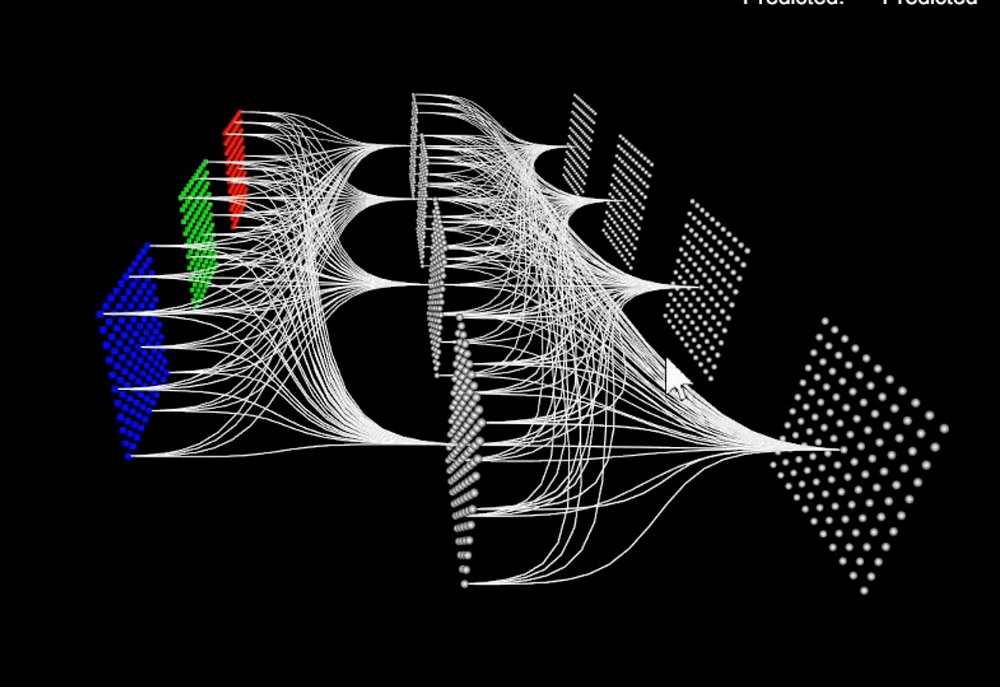# Talented Mr. 1X1: Comprehensive look at 1X1 Convolution in Deep Learning

Source: Deep Learning on Medium# Talented Mr. 1X1: Comprehensive look at 1X1 Convolution in Deep Learning

With startling success of AlexNet in 2012, the Convolutional Neural Net (CNN) revolution has begun! The CNN based frameworks in Deep Learning like GoogleNet, ResNet and several variations of these have shown spectacular results in the object detection and semantic segmentation in computer vision.

When you start to look at most of the successful modern CNN architectures, like GoogleNet, ResNet and SqueezeNet you will come across 1X1 Convolution layer playing a major role. At first glance, it seems to be pointless to employ a single digit to convolve with the input image (After all wider filters like 3X3, 5X5 can work on a patch of image as opposed to a single pixel in this case). However, 1X1 convolution has proven to be extremely useful tool and employed correctly, will be instrumental in creating wonderfully deep architectures.

In this article we will have a detailed look at 1X1 Convolutions

First a quick recap of Convolutions in Deep Learning. There are many good blogs and articles that intuitively explain what convolutions are and different types of convolutions (few of them are listed in the reference). While we will not delve deep into the convolutions in this article, understanding couple of key points will make it easier to get what 1X1 convolution is doing and most importantly How & Why it is doing it.

## Quick Recap: Convolution in Deep Learning

As mentioned, this article will not provide a complete treatment of theory and practice of Convolution. However, we will recap key principles of Convolution in deep learning. This will come in handy when we examine 1X1 Convolution in depth.

Simply put, Convolutions is an element wise multiplication and summation of the input and kernel/filter elements. Now the data points to remember

1. Input matrix can and, in most cases, will have more than one channel. This is sometimes referred to as depth

a. Example: 64X64 pixel RGB input from an image will have 3 channels so the input is 64X64X3

2. The filter has the same depth as input except in some special cases (example 3D Convolutions to reconstruct medical images). This specific point, for some unknown reason, is not explicitly mentioned in most of the literature, causing some misunderstanding (Especially for someone new to convolutions, Deep learning etc)

a. Example: filter of 3X3 will have 3 channels as well, hence the filter should be represented as 3X3X3

3. Third and critical point, the output of Convolution step will have the depth equal to number of filters we choose.

a. Example: Output of Convolution step of the 3D input (64X64X3) and the filter we chose (3X3X3) will have the depth of 1 (Because we have only one filter)

The Convolution step on the 3D input 64X64X3 with filter size of 3X3X3 will have the filter ‘sliding’ along the width and height of the input.

So, when we convolve the 3D filter with the 3D image, the operation moves the filter on the input in 2 directions (Along the width and height) and we do the element wise multiplication and addition at each position to end up with an output with a depth of 1.

Armed with this, we are ready to dive into the 1X1 convolution

## 1X1 Convolution — What is it?

Introduced first in a paper by Min Lin et all in their Network In Network, the 1X1 Convolution layer was used for ‘Cross Channel Down sampling’ or Cross Channel Pooling. In other words, 1X1 Conv was used to reduce the number of channels while introducing non-linearity.

In 1X1 Convolution simply means the filter is of size 1X1 (Yes — that means a single number as opposed to matrix like, say 3X3 filter). This 1X1 filter will convolve over the ENTIRE input image pixel by pixel.

Staying with our example input of 64X64X3, if we choose a 1X1 filter (which would be 1X1X3), then the output will have the same Height and Weight as input but only one channel — 64X64X1

Now consider inputs with large number of channels — 192 for example. If we want to reduce the depth and but keep the Height X Width of the feature maps (Receptive field) the same, then we can choose 1X1 filters (remember Number of filters = Output Channels) to achieve this effect. This effect of cross channel down-sampling is called ‘Dimensionality reduction’.

Now why would we want to something like that? For that we delve into usage of 1X1 Convolution

Usage 1: Dimensionality Reduction/Augmentation

Winner of ILSVRC (ImageNet Large Scale Visual Recognition Competition) 2014, GoogleNet, used 1X1 convolution layer for dimension reduction “to compute reductions before the expensive 3×3 and 5×5 convolutions”

Let us look at an example to understand how reducing dimension will reduce computational load. Suppose we need to convolve 28 X 28 X 192 input feature maps with 5 X 5 X 32 filters. This will result in 120.422 Million operations